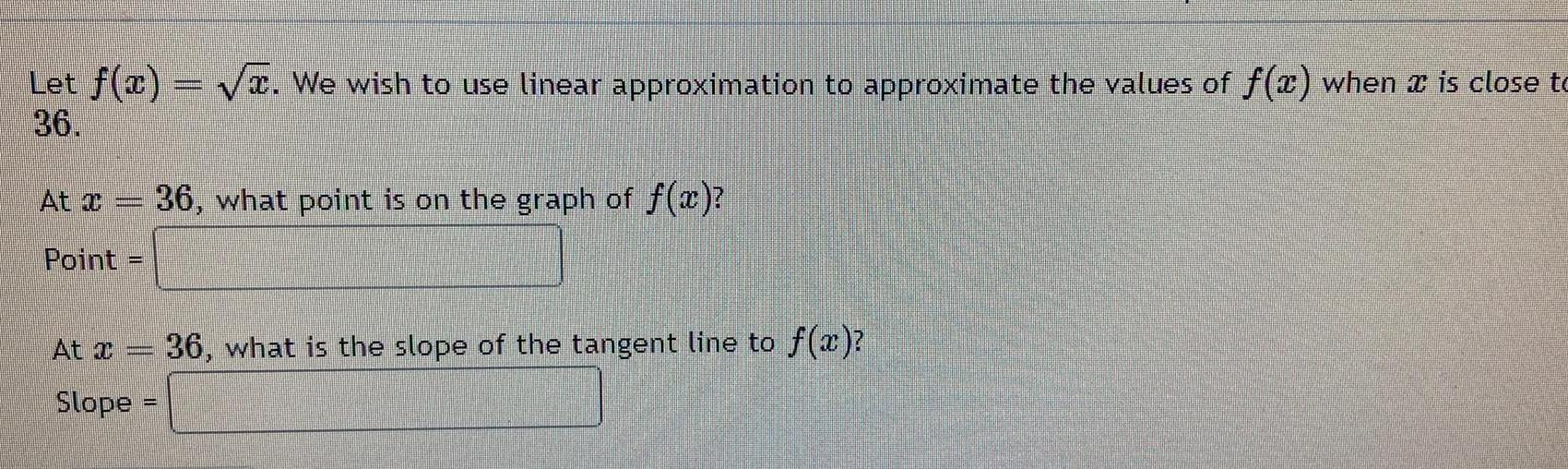Question:

# Let f x We wish to use linear approximation to approximate

Last updated: 9/19/2023Let f x We wish to use linear approximation to approximate the values of f x when is close to 36 At x 36 what point is on the graph of f x Point At Jumanni Comm Slope 36 what is the slope of the tangent line to f x